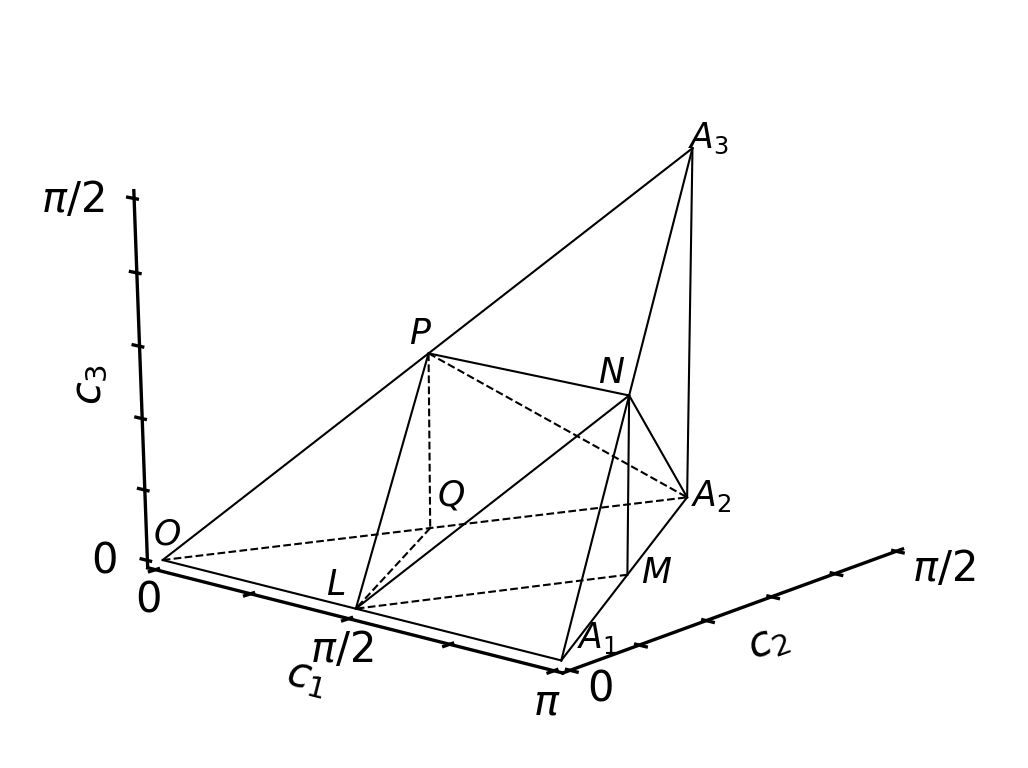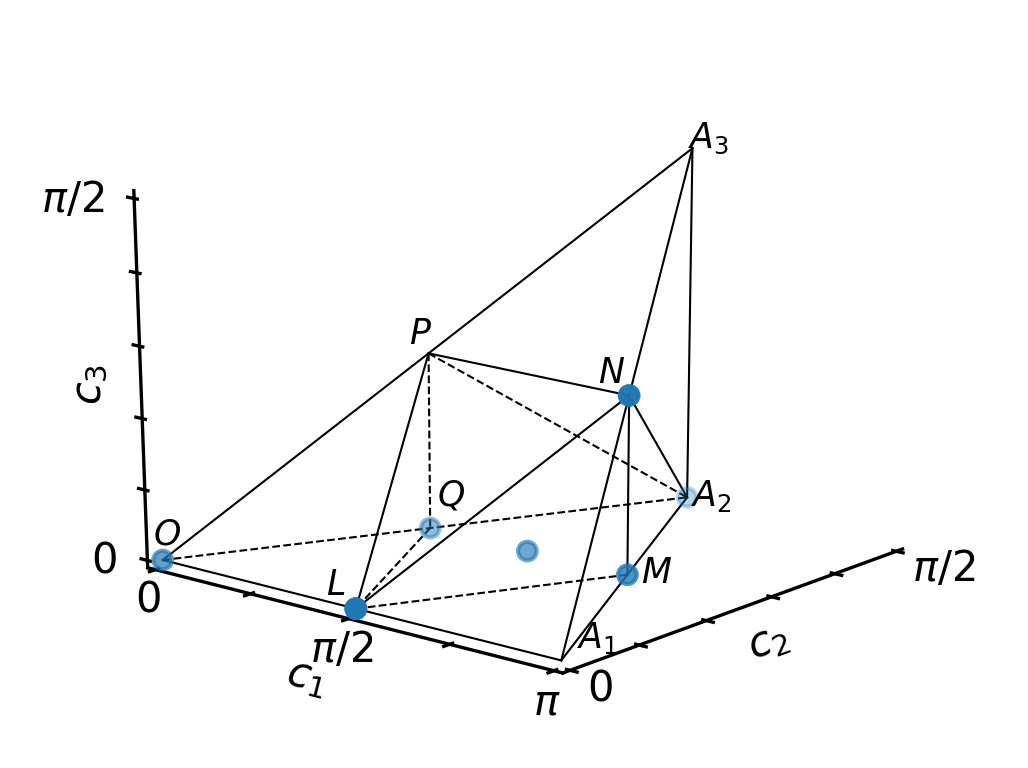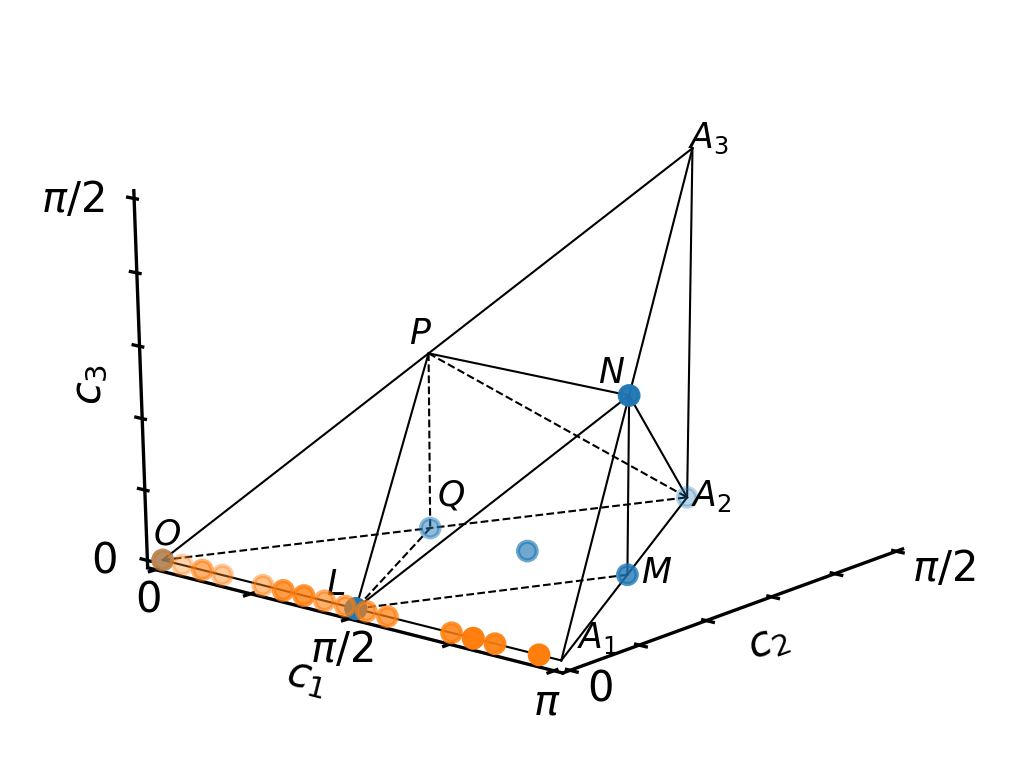Tutorial¶

In :
# NBVAL_IGNORE_OUTPUT
import numpy as np
import qutip
import matplotlib
import matplotlib.pylab as plt
import weylchamber
from weylchamber.visualize import WeylChamber
%watermark -v --iversions
numpy       1.15.4
qutip       4.3.1
matplotlib  3.0.2
matplotlib.pylab  1.15.4
weylchamber  0.1.0
CPython 3.6.7
IPython 7.1.1

$\newcommand{Re}{\operatorname{Re}} \newcommand{Im}{\operatorname{Im}} \newcommand{dd}{\,\text{d}} \newcommand{abs}{\operatorname{abs}}$

Every two-qubit gate is associated with a point in the "Weyl-chamber" that may be visualized in three dimensions as the following polyhedron:

In :
WeylChamber().plot()Note: if you run this interactively, and switch to an interactive matplotlib backend, e.g.

%matplotlib tk

you will be able to rotate the 3D plot to get a better intuition.

Consider the following common two-qubit gates:

In :
IDENTITY = qutip.gates.identity([2,2])
IDENTITY
Out:
Quantum object: dims = [[2, 2], [2, 2]], shape = (4, 4), type = oper, isherm = True\begin{equation*}\left(\begin{array}{*{11}c}1.0 & 0.0 & 0.0 & 0.0\\0.0 & 1.0 & 0.0 & 0.0\\0.0 & 0.0 & 1.0 & 0.0\\0.0 & 0.0 & 0.0 & 1.0\\\end{array}\right)\end{equation*}
In :
CNOT = qutip.gates.cnot()
CNOT
Out:
Quantum object: dims = [[2, 2], [2, 2]], shape = (4, 4), type = oper, isherm = True\begin{equation*}\left(\begin{array}{*{11}c}1.0 & 0.0 & 0.0 & 0.0\\0.0 & 1.0 & 0.0 & 0.0\\0.0 & 0.0 & 0.0 & 1.0\\0.0 & 0.0 & 1.0 & 0.0\\\end{array}\right)\end{equation*}
In :
CPHASE = qutip.gates.cphase(np.pi)
CPHASE
Out:
Quantum object: dims = [[2, 2], [2, 2]], shape = (4, 4), type = oper, isherm = True\begin{equation*}\left(\begin{array}{*{11}c}1.0 & 0.0 & 0.0 & 0.0\\0.0 & 1.0 & 0.0 & 0.0\\0.0 & 0.0 & 1.0 & 0.0\\0.0 & 0.0 & 0.0 & -1.0\\\end{array}\right)\end{equation*}
In :
BGATE = qutip.gates.berkeley()
BGATE
Out:
Quantum object: dims = [[2, 2], [2, 2]], shape = (4, 4), type = oper, isherm = False\begin{equation*}\left(\begin{array}{*{11}c}0.924 & 0.0 & 0.0 & 0.383j\\0.0 & 0.383 & 0.924j & 0.0\\0.0 & 0.924j & 0.383 & 0.0\\0.383j & 0.0 & 0.0 & 0.924\\\end{array}\right)\end{equation*}
In :
iSWAP = qutip.gates.iswap()
iSWAP
Out:
Quantum object: dims = [[2, 2], [2, 2]], shape = (4, 4), type = oper, isherm = False\begin{equation*}\left(\begin{array}{*{11}c}1.0 & 0.0 & 0.0 & 0.0\\0.0 & 0.0 & 1.0j & 0.0\\0.0 & 1.0j & 0.0 & 0.0\\0.0 & 0.0 & 0.0 & 1.0\\\end{array}\right)\end{equation*}
In :
sqrtISWAP = qutip.gates.sqrtiswap()
sqrtISWAP
Out:
Quantum object: dims = [[2, 2], [2, 2]], shape = (4, 4), type = oper, isherm = False\begin{equation*}\left(\begin{array}{*{11}c}1.0 & 0.0 & 0.0 & 0.0\\0.0 & 0.707 & 0.707j & 0.0\\0.0 & 0.707j & 0.707 & 0.0\\0.0 & 0.0 & 0.0 & 1.0\\\end{array}\right)\end{equation*}
In :
sqrtSWAP = qutip.gates.sqrtswap()
sqrtSWAP
Out:
Quantum object: dims = [[2, 2], [2, 2]], shape = (4, 4), type = oper, isherm = False\begin{equation*}\left(\begin{array}{*{11}c}1.0 & 0.0 & 0.0 & 0.0\\0.0 & (0.500+0.500j) & (0.500-0.500j) & 0.0\\0.0 & (0.500-0.500j) & (0.500+0.500j) & 0.0\\0.0 & 0.0 & 0.0 & 1.0\\\end{array}\right)\end{equation*}
In :
MGATE = weylchamber.canonical_gate(3/4, 1/4, 0)
MGATE
Out:
Quantum object: dims = [[2, 2], [2, 2]], shape = (4, 4), type = oper, isherm = False\begin{equation*}\left(\begin{array}{*{11}c}0.707 & 0.0 & 0.0 & 0.707j\\0.0 & 0.0 & 1.0j & 0.0\\0.0 & 1.0j & 0.0 & 0.0\\0.707j & 0.0 & 0.0 & 0.707\\\end{array}\right)\end{equation*}

All of these gates are situatated at special points in the Weyl chamber. We can print their Weyl chamber coordinates and add a point in the graphical representation

In :
w = WeylChamber();
list_of_gates = [
('Identity', IDENTITY),
('CNOT', CNOT), ('CPHASE', CPHASE), ('BGATE', BGATE),
('iSWAP', iSWAP), ('sqrtISWAP', sqrtISWAP),
('sqrtSWAP', sqrtSWAP), ('MGATE', MGATE)]
print("Weyl Chamber Coordinates")
print("----------------------------------")
for (name, gate) in list_of_gates:
c1, c2, c3 = weylchamber.c1c2c3(gate)
print("%10s: \t%.2fπ %.2fπ %.2fπ" % (name, c1, c2, c3))
w.plot()
Weyl Chamber Coordinates
----------------------------------
Identity: 	0.00π 0.00π 0.00π
CNOT: 	0.50π 0.00π 0.00π
CPHASE: 	0.50π 0.00π 0.00π
BGATE: 	0.50π 0.25π 0.00π
iSWAP: 	0.50π 0.50π 0.00π
sqrtISWAP: 	0.25π 0.25π 0.00π
sqrtSWAP: 	0.75π 0.25π 0.25π
MGATE: 	0.75π 0.25π 0.00πThe gates locally equivalent to the controlled-phase gates are on an the axis 0 - A1 in the Weyl chamber:

In :
w.scatter(*zip(*[
weylchamber.c1c2c3(qutip.gates.cphase(phase))
for phase in np.linspace(0, 2*np.pi, 20)]))
In :
w.plot()The Weyl chamber coordinates $(c_1, c_2, c_3)$ are closely associated with the local invariants $(g_1, g_2, g_3)$

In :
print("Local Invariants")
print("----------------------------------")
for (name, gate) in list_of_gates:
g1, g2, g3 = weylchamber.g1g2g3(gate)
print("%10s: \t%5.2f %5.2f %5.2f" % (name, g1, g2, g3))
Local Invariants
----------------------------------
Identity: 	 1.00  0.00  3.00
CNOT: 	 0.00  0.00  1.00
CPHASE: 	 0.00  0.00  1.00
BGATE: 	 0.00 -0.00  0.00
iSWAP: 	 0.00  0.00 -1.00
sqrtISWAP: 	 0.25  0.00  1.00
sqrtSWAP: 	 0.00 -0.25  0.00
MGATE: 	 0.25  0.00  1.00

This shows that the MGATE and $\sqrt{\text{iSWAP}}$ are actually locally equivalent, despite being different Weyl chamber coordinates (M and Q, respectively)